Courses
Courses for Kids
Free study material
Offline Centres
More

# NCERT Solutions for Class 12 Maths Chapter 8 - ExerciseLast updated date: 05th Dec 2023
Total views: 534.9k
Views today: 8.34k

## NCERT Solutions for Class 12 Maths Chapter 8 Application of Integrals (Ex 8.2) Exercise 8.2

Free PDF download of NCERT Solutions for Class 12 Maths Chapter 8 Exercise 8.2 (Ex 8.2) and all chapter exercises at one place prepared by expert teacher as per NCERT (CBSE) books guidelines. Class 12 Maths Chapter 8 Application of Integrals Exercise 8.2 Questions with Solutions to help you to revise complete Syllabus and Score More marks. Register and get all exercise solutions in your emails.

## Access NCERT Solution for Class 12 Maths Chapter 8 – Application of Integrals

Exercise 8.2

1. Find the area of the circle$4{x^2} + 4{y^2} = 9$ which is interior to the parabola${x^2} = 4y$.

Ans: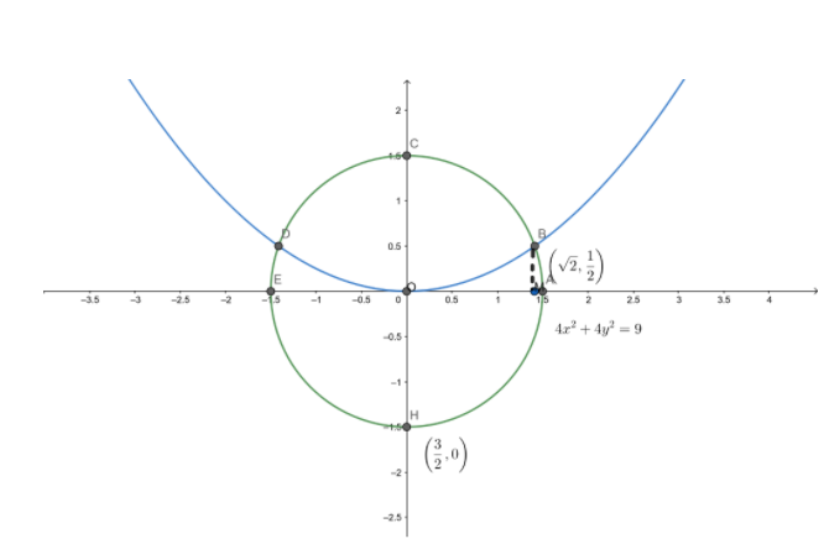Solving the given equation of circle, $4{x^2} + 4{y^2} = 9$and the parabola ${x^2} = 4y$

$4\left( {4y} \right) + 4{y^2} = 9$

$4{y^2} + 16y - 9 = 0$

$y = \dfrac{{ - 16 \pm \sqrt {{{16}^2} - 4 \times 4 \times \left( { - 9} \right)} }}{{2 \times 4}}$

$y = \dfrac{1}{2}{\text{ or }} - 4.5$

The value of $y$ cannot be negative as ${x^2} = 4y$

We obtain the point of intersection as $B\left( {\sqrt 2 ,\dfrac{1}{2}} \right)$ and$D\left( { - \sqrt 2 ,\dfrac{1}{2}} \right)$

It can be observed that the required area is symmetrical about y-axis.

$\therefore {\text{Area OBCDO = 2 . Area OBCO}}$

We draw ${\text{BM}}$ perpendicular to ${\text{OA}}$.

Therefore, the coordinates of ${\text{M}}$are $\left( {\sqrt 2 ,0} \right)$,

Therefore, ${\text{Area OBCO = Area OMBCO -- Area OMBO}}$

${\text{Area OMBCO}} =$Area under the circle $4{x^2} + 4{y^2} = 9$ between $x = 0$and $x = \sqrt 2$

${\text{Area OMBCO}}$= $\int\limits_0^{\sqrt 2 } {\sqrt {\dfrac{{9 - 4{x^2}}}{4}} dx}$

$= \int\limits_0^{\sqrt 2 } {\sqrt {\dfrac{{9 - 4{x^2}}}{4}} dx}$

Substitute $x = \dfrac{3}{2}\sin \theta$,$dx = \dfrac{3}{2}\cos \theta d\theta$

then,

$\int {\sqrt {\dfrac{9}{4} - \dfrac{9}{4}{{\sin }^2}\theta } \dfrac{3}{2}\cos \theta d\theta }$

$= \dfrac{9}{4}\int {\sqrt {1 - {{\sin }^2}\theta } \cos \theta d\theta }$

$= \dfrac{9}{4}\int {{{\cos }^2}\theta d\theta }$

$= \dfrac{9}{4}\int {\dfrac{{1 + \cos 2\theta }}{2}d\theta }$

$= \dfrac{9}{4}\int {\dfrac{1}{2} + \dfrac{{\cos 2\theta }}{2}d\theta }$

$= \dfrac{9}{8}\left[ {\theta + \dfrac{{\sin 2\theta }}{2}} \right]$

$= \dfrac{9}{8}\left[ {\theta + \dfrac{{2\sin \theta \cos \theta }}{2}} \right]$

$= \dfrac{9}{8}\left[ {{{\sin }^{ - 1}}\dfrac{{2x}}{3} + \dfrac{{2x}}{3}\sqrt {1 - \dfrac{{4{x^2}}}{9}} } \right]$

Applying the limits, then

$= \dfrac{9}{8}\left[ {{{\sin }^{ - 1}}\dfrac{{2x}}{3} + \dfrac{{2x}}{9}\sqrt {4{x^2} - 9} } \right]_0^{\sqrt 2 }$

$= \dfrac{9}{8}\left[ {{{\sin }^{ - 1}}\dfrac{{2\sqrt 2 }}{3} + \dfrac{{2\sqrt 2 }}{9}\sqrt {4 \times 2 - 9} - 0} \right]$

${\text{Area OMBCO}}$$= \dfrac{1}{4}\left[ {\sqrt 2 + \dfrac{9}{2}{{\sin }^{ - 1}}\dfrac{{2\sqrt 2 }}{3}} \right]$…………….. (1)

${\text{Area OMBCO}}$${\text{ = }}$Area under between${x^2} = 4y$and$x = 0$and$x = \sqrt 2$

${\text{Area OMBO}}$$= \int\limits_0^{\sqrt 2 } {\dfrac{{{x^2}}}{4}}$

$= \dfrac{1}{4}\left[ {\dfrac{{{x^3}}}{3}} \right]_0^{\sqrt 2 }$

${\text{Area OMBO}}$$= \dfrac{1}{{12}}\left[ {2\sqrt 2 } \right] = \dfrac{{\sqrt 2 }}{6}$…………… (2)

From (1) and (2)

${\text{Area OBCO }}$$= \dfrac{{\sqrt 2 }}{2} + \dfrac{9}{8}{\sin ^{ - 1}}\dfrac{{2\sqrt 2 }}{3} - \dfrac{{\sqrt 2 }}{6}$

$= \dfrac{{\sqrt 2 }}{{12}} + \dfrac{9}{8}{\sin ^{ - 1}}\dfrac{{2\sqrt 2 }}{3}$

$= \dfrac{1}{2}\left( {\dfrac{{\sqrt 2 }}{6} + \dfrac{9}{4}{{\sin }^{ - 1}}\dfrac{{2\sqrt 2 }}{3}} \right)$

Then the required area ${\text{ OBCDO}}$ is$= 2 \times \dfrac{1}{2}\left( {\dfrac{{\sqrt 2 }}{6} + \dfrac{9}{4}{{\sin }^{ - 1}}\dfrac{{2\sqrt 2 }}{3}} \right)$

$= \dfrac{{\sqrt 2 }}{6} + \dfrac{9}{4}{\sin ^{ - 1}}\dfrac{{2\sqrt 2 }}{3}sq.units$

2. Find the area bounded by curves ${\left( {x - 1} \right)^2} + {y^2} = 1$and ${x^2} + {y^2} = 1$

Ans: The are bounded by the curves, ${\left( {x - 1} \right)^2} + {y^2} = 1$and${x^2} + {y^2} = 1$, is represented by the shaded area as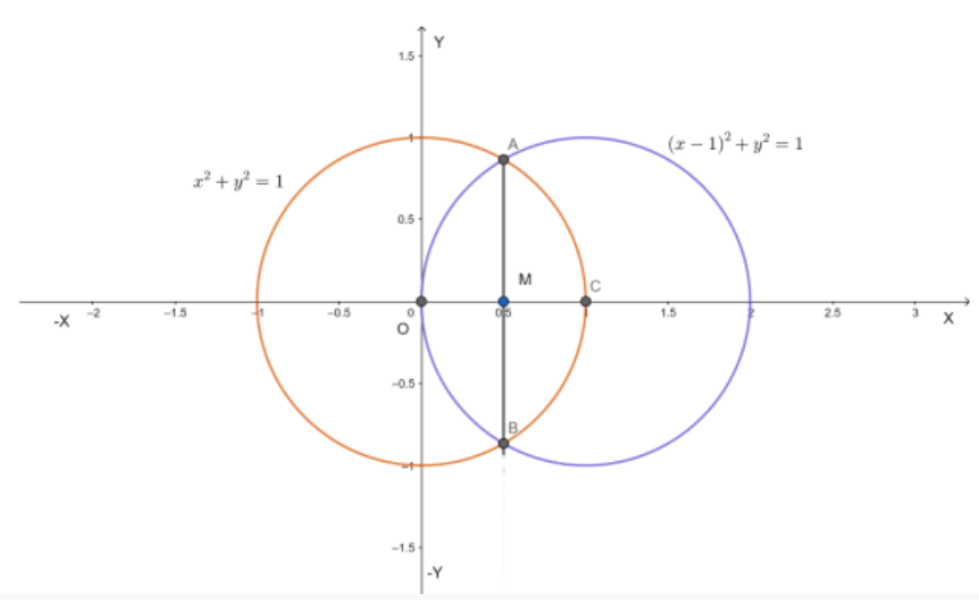On solving the equations,${\left( {x - 1} \right)^2} + {y^2} = 1$ and ${x^2} + {y^2} = 1$

${\left( {x - 1} \right)^2} + 1 - {x^2} = 1$

$- 2x + 1 = 0$

$x = \dfrac{1}{2}$

${y^2} = 1 - {x^2}$

$= 1 - \dfrac{1}{4} = \dfrac{3}{4}$

we obtain the point of intersection as $A\left( {\dfrac{1}{2},\dfrac{{\sqrt 3 }}{2}} \right)$and$B\left( {\dfrac{1}{2}, - \dfrac{{\sqrt 3 }}{2}} \right)$

It can be observed that the required area is symmetrical about x-axis.

$\therefore {\text{ Area OBCAO = }}2 \times {\text{Area OCAO}}$

We join ${\text{AB}}$ which intersects ${\text{OC}}$at ${\text{M}}$, such that ${\text{AM}}$ is perpendicular to ${\text{OC}}$.

The coordinates of M are$\left( {\dfrac{1}{2},0} \right)$.

$\Rightarrow {\text{ Area OCAO = Area OMAO}} + {\text{Area MCAM}}$

$= \int\limits_0^{\dfrac{1}{2}} {\sqrt {1 - {{\left( {x - 1} \right)}^2}} dx + } \int\limits_{\dfrac{1}{2}}^1 {\sqrt {1 - {x^2}} dx}$

$= \left[ {\dfrac{{x - 1}}{2}\sqrt {1 - {{\left( {x - 1} \right)}^2}} + \dfrac{1}{2}{{\sin }^{ - 1}}\left( {x - 1} \right)} \right]_0^{\dfrac{1}{2}} + \left[ {\dfrac{x}{2}\sqrt {1 - {x^2}} + \dfrac{1}{2}{{\sin }^{ - 1}}x} \right]_{\dfrac{1}{2}}^1$

$= \left[ {\dfrac{{ - 1}}{4}\sqrt {1 - {{\left( { - \dfrac{1}{2}} \right)}^2}} + \dfrac{1}{2}{{\sin }^{ - 1}}\left( {\dfrac{1}{2} - 1} \right) - \dfrac{1}{2}{{\sin }^{ - 1}}\left( { - 1} \right)} \right]_0^{\dfrac{1}{2}} + \left[ {\dfrac{1}{2}{{\sin }^{ - 1}}\left( 1 \right) - \dfrac{1}{4}\sqrt {1 - {{\left( {\dfrac{1}{2}} \right)}^2}} - \dfrac{1}{2}{{\sin }^{ - 1}}\left( {\dfrac{1}{2}} \right)} \right]_{\dfrac{1}{2}}^1$

$= \left[ { - \dfrac{{\sqrt 3 }}{8} + \dfrac{1}{2}\left( { - \dfrac{\pi }{6}} \right) - \dfrac{1}{2}\left( { - \dfrac{\pi }{2}} \right)} \right] + \left[ {\dfrac{1}{2}\left( {\dfrac{\pi }{2}} \right) - \dfrac{{\sqrt 3 }}{8} - \dfrac{1}{2}\left( {\dfrac{\pi }{6}} \right)} \right]$

$= - \dfrac{{\sqrt 3 }}{4} - \dfrac{\pi }{{12}} + \dfrac{\pi }{4} + \dfrac{\pi }{4} - \dfrac{\pi }{{12}}$

$= - \dfrac{{\sqrt 3 }}{4} + \dfrac{{2\pi }}{6}$

Therefore, required area ${\text{OBCAO}}$$= 2 \times \left( {\dfrac{{2\pi }}{6} - \dfrac{{\sqrt 3 }}{4}} \right)$

$= \left( {\dfrac{{2\pi }}{3} - \dfrac{{\sqrt 3 }}{2}} \right)sq.units$

3. Find the area of the region bounded by the curves$y = {x^2} + 2$,$y = x$,$x = 0$ and$x = 3$.

Ans: The area bounded by the curves,$y = {x^2} + 2$,$y = x$,$x = 0$ and $x = 3$, is represented by the area ${\text{OCBAO}}$as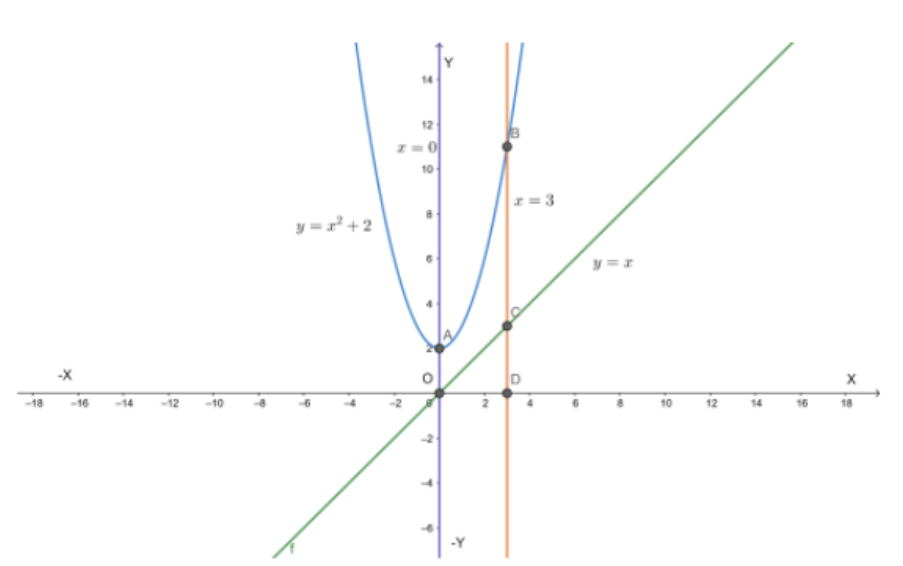Then, ${\text{Area OCBAO = Area ODBAO -- Area ODCO}}$

$= \int\limits_0^3 {\left( {{x^2} + 2} \right)dx} - \int\limits_0^3 {xdx}$

$= \left[ {\dfrac{{{x^3}}}{3} + 2x} \right]_0^3 - \left[ {\dfrac{{{x^2}}}{2}} \right]_0^3$

$= \left[ {9 + 6} \right] - \left[ {\dfrac{9}{2}} \right]$

$= 15 - \dfrac{9}{2}$

Therefore, required area$= \dfrac{{21}}{2}sq.units$

4. Using integration find the area of the region bounded by the triangle whose vertices are$\left( { - 1,0} \right)$,$\left( {1,3} \right)$ and$\left( {3,2} \right)$.

Ans: BL and CM are drawn perpendicular to x-axis.

It can be observed in the following figure that,

${\text{Area}}\left( {\Delta {\text{ACB}}} \right) = {\text{Area}}\left( {{\text{ALBA}}} \right) + {\text{Area}}\left( {{\text{BLMCB}}} \right){\text{ - Area}}\left( {{\text{AMCA}}} \right)$………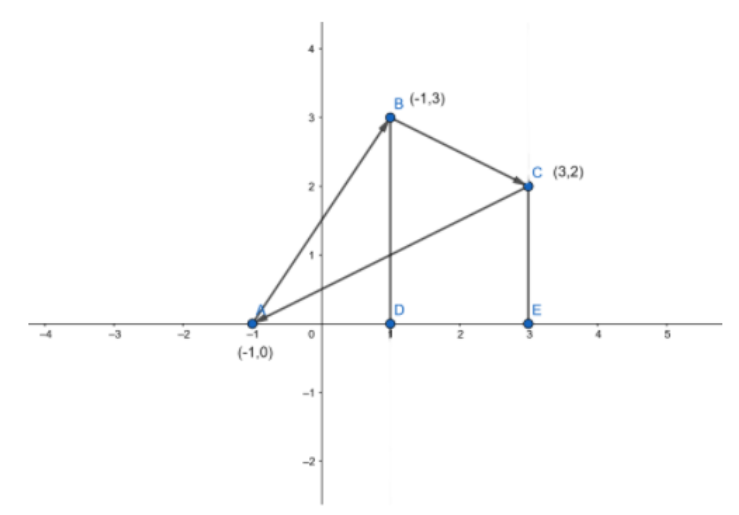Equation of line segment ${\text{AB}}$is

${\text{y - 0 = }}\dfrac{{3 - 0}}{{1 + 1}}\left( {x + 1} \right)$

$y = \dfrac{3}{2}\left( {x + 1} \right)$

$\therefore {\text{Area}}\left( {{\text{ALBA}}} \right) = \int\limits_{ - 1}^1 {\dfrac{3}{2}\left( {x + 1} \right)dx} = \dfrac{3}{2}\left[ {\dfrac{{{x^2}}}{2} + x} \right]_{ - 1}^1$

$= \dfrac{3}{2}\left[ {\dfrac{1}{2} + 1 - \dfrac{1}{2} + 1} \right] = 3{\text{ }}sq.units$

Equation of line segment ${\text{BC}}$ is

${\text{y - 3 = }}\dfrac{{2 - 3}}{{3 - 1}}\left( {x - 1} \right)$

$y = \dfrac{1}{2}\left( { - x + 7} \right)$

$\therefore {\text{Area}}\left( {{\text{BLMCB}}} \right) = \int\limits_1^3 {\dfrac{1}{2}\left( { - x + 7} \right)dx} = \dfrac{1}{2}\left[ { - \dfrac{{{x^2}}}{2} + 7x} \right]_1^3$

$= \dfrac{1}{2}\left[ { - \dfrac{9}{2} + 21 + \dfrac{1}{2} - 7} \right] = 5{\text{ }}sq.units$

Equation of line segment ${\text{AC}}$is

${\text{y - 0 = }}\dfrac{{2 - 0}}{{3 + 1}}\left( {x + 1} \right)$

$y = \dfrac{1}{2}\left( {x + 1} \right)$

$\therefore {\text{Area}}\left( {{\text{AMCA}}} \right) = \int\limits_{ - 1}^3 {\dfrac{1}{2}\left( {x + 1} \right)dx} = \dfrac{1}{2}\left[ {\dfrac{{{x^2}}}{2} + x} \right]_{ - 1}^3$

$= \dfrac{1}{2}\left[ {\dfrac{9}{2} + 3 - \dfrac{1}{2} + 1} \right] = 4{\text{ }}sq.units$

Therefore, From equation,we obtain

${\text{Area}}\left( {\Delta {\text{ACB}}} \right) = 3 + 5 - 4 = 4{\text{ }}sq.units$

5. Using integration find the area of the triangular region whose sides have the equations $y = 2x + 1$,$y = 3x + 1$and$x = 4$.

Ans:The equations of sides of the triangle are $y = 2x + 1$,$y = 3x + 1$and $x = 4$.

On solving these equations, we obtain the vertices of triangle as ${\text{A}}\left( {0,1} \right)$,${\text{B}}\left( {4,13} \right)$and ${\text{C}}\left( {4,9} \right)$.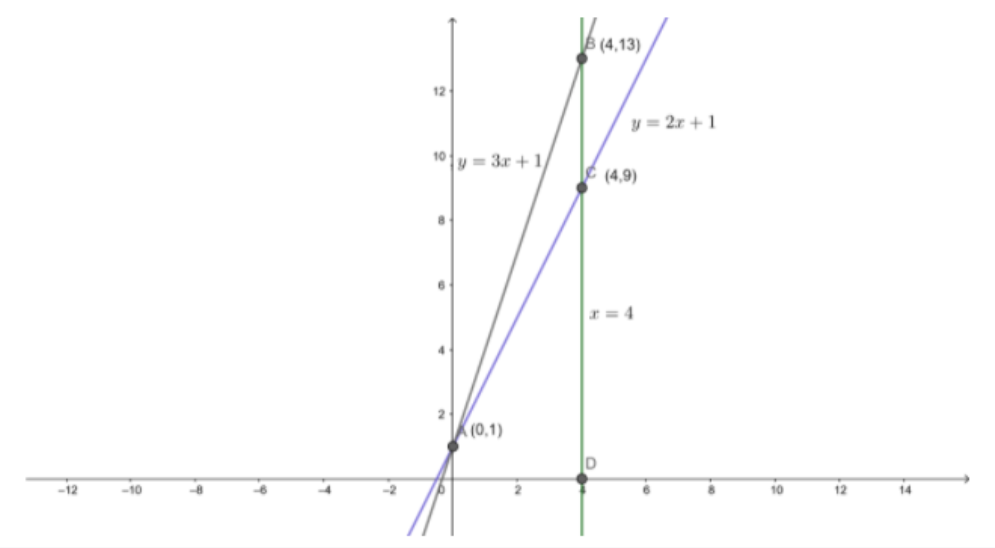It can be observed that,

${\text{Area }}\left( {\Delta {\text{ACB}}} \right) = {\text{Area }}\left( {{\text{OLBAO}}} \right) - {\text{Area}}\left( {{\text{OLCAO}}} \right)$

$= \int\limits_0^4 {\left( {3x + 1} \right)dx} - \int\limits_0^4 {\left( {2x + 1} \right)dx}$

$= \left[ {\dfrac{{3{x^2}}}{2} + x} \right]_0^4 - \left[ {\dfrac{{2{x^2}}}{2} + x} \right]_0^4$

$= \left( {24 + 4} \right) - \left( {16 + 4} \right)$

$= 28 - 20$

Therefore, required area $= 8{\text{ }}sq.units$

6. Smaller area enclosed by the circle ${x^2} + {y^2} = 4$and the line $x + y = 2$is

(A)  $2\left( {\pi - 2} \right)$(B)$\pi - 2$   (C) $2\pi - 1$  (D)$2\left( {\pi + 2} \right)$

Ans: The smaller area enclosed by the circle${x^2} + {y^2} = 4$ and the line$x + y = 2$, is represented by the area ${\text{ACBA}}$ as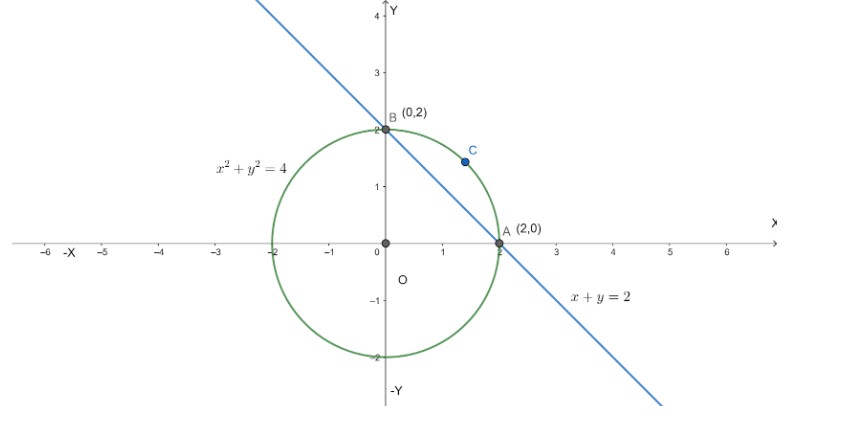It can be observed that

${\text{Area }}\left( {{\text{ACBA}}} \right) = {\text{Area }}\left( {{\text{OACBO}}} \right) - {\text{Area}}\left( {\Delta {\text{OAB}}} \right)$

$= \int\limits_0^2 {\sqrt {4 - {x^2}} dx} - \int\limits_0^2 {\left( {2 - x} \right)dx}$

$= \left[ {\dfrac{x}{2}\sqrt {4 - {x^2}} + \dfrac{4}{2}{{\sin }^{ - 1}}\dfrac{x}{2}} \right]_0^2 - \left[ {2x - \dfrac{{{x^2}}}{2}} \right]_0^2$

$= \left[ {2\dfrac{\pi }{2}} \right] - \left[ {4 - 2} \right]$

$= \left( {\pi - 2} \right){\text{ }}sq.units$

Thus, the correct answer is ${\text{B}}$.

7. Area lying between the curves ${y^2} = 4x$and$y = 2x$ is

(A)$\dfrac{2}{3}$  (B)  $\dfrac{1}{3}$ (C) $\dfrac{1}{4}$  (D)$\dfrac{3}{4}$

Ans:The area lying between the curves${y^2} = 4x$and $y = 2x$, is represented by the shaded area ${\text{OABO}}$ as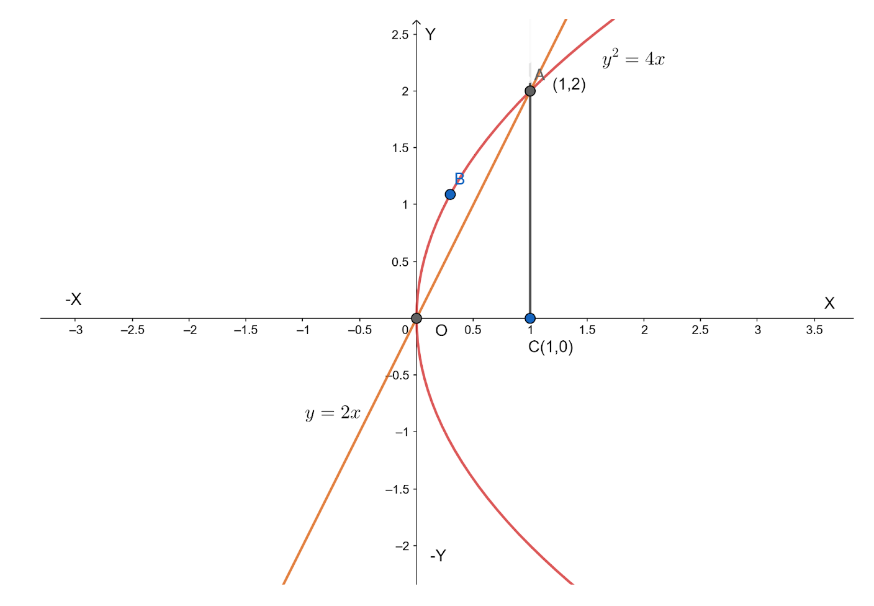The points of intersection of these curves are ${\text{O}}\left( {0,0} \right)$ and ${\text{A}}\left( {1,2} \right)$

We draw ${\text{AC}}$ perpendicular to $x{\text{ - axis}}$ such that the coordinates of ${\text{C}}$ are $\left( {1,0} \right)$

${\text{Area }}\left( {{\text{OBAO}}} \right) = {\text{Area }}\left( {\Delta {\text{OCA}}} \right) - {\text{Area}}\left( {{\text{OCABO}}} \right)$

$= \int\limits_0^1 {2xdx} - \int\limits_0^1 {2\sqrt x dx}$

$= 2\left[ {\dfrac{{{x^2}}}{2}} \right]_0^1 - 2\left[ {\dfrac{{{x^{\dfrac{3}{2}}}}}{{\dfrac{3}{2}}}} \right]_0^1$

$= \left| {1 - \dfrac{4}{3}} \right|$

$= \left| { - \dfrac{1}{3}} \right|$

$= \dfrac{1}{3}{\text{ }}$sq.units

Thus, the correct answer is ${\text{B}}$.

### NCERT Solution Class 12 Maths of Chapter 8 All Exercises

 Chapter 8 - Application of Integrals Exercises in PDF Format Exercise 8.1 13 Questions & Solutions (11 Short Answers, 2 MCQs) 7 Questions & Solutions (5 Short Answers, 2 MCQs)

## NCERT Solutions for Class 12 Maths Chapter 8 Application of Integrals Exercise 8.2

Opting for the NCERT solutions for Ex 8.2 Class 12 Maths is considered as the best option for the CBSE students when it comes to exam preparation. This chapter consists of many exercises. Out of which we have provided the Exercise 8.2 Class 12 Maths NCERT solutions on this page in PDF format. You can download this solution as per your convenience or you can study it directly from our website/ app online.

Vedantu in-house subject matter experts have solved the problems/ questions from the exercise with the utmost care and by following all the guidelines by CBSE. Class 12 students who are thorough with all the concepts from the Maths textbook and quite well-versed with all the problems from the exercises given in it, then any student can easily score the highest possible marks in the final exam. With the help of this Class 12 Maths Chapter 8 Exercise 8.2 solutions, students can easily understand the pattern of questions that can be asked in the exam from this chapter and also learn the marks weightage of the chapter. So that they can prepare themselves accordingly for the final exam.

Besides these NCERT solutions for Class 12 Maths Chapter 8 Exercise 8.2, there are plenty of exercises in this chapter which contain innumerable questions as well. All these questions are solved/answered by our in-house subject experts as mentioned earlier. Hence all of these are bound to be of superior quality and anyone can refer to these during the time of exam preparation. In order to score the best possible marks in the class, it is really important to understand all the concepts of the textbooks and solve the problems from the exercises given next to it.

Do not delay any more. Download the NCERT solutions for Class 12 Maths Chapter 8 Exercise 8.2 from Vedantu website now for better exam preparation. If you have the Vedantu app in your phone, you can download the same through the app as well. The best part of these solutions is these can be accessed both online and offline as well.

## FAQs on NCERT Solutions for Class 12 Maths Chapter 8 - Exercise

1. Which formula to use to calculate the region with curve y = f (x), where x-axis and the lines x = a and x = b (b > a) ?

In order to calculate the region which is bounded by the curve y = f (x), where x-axis and the lines x = a and x = b (b > a), you will use the formula ∫ba ydx =  ∫ba f (x)dx. To have a better comprehension of the exercise, use Vedantu’s NCERT Solutions Class 12 Maths Chapter 8 Exercise 8.2. It is comprehensive as it touches each and every important aspect of the questions - from stepwise solutions to the application of formulas.

2. How many questions are there in Exercise 8.2 of Chapter 8 of Class 12 Maths?

There are seven important questions in total in Exercise 8.2 of Chapter 8 of Class 12 Maths. The questions are essentially based on the area between two curves. To solve this exercise smoothly, you must consider referring to NCERT Solutions of  Exercise 8.2 of Chapter 8 of Class 12 Maths crafted by the experts at Vedantu. These expert solutions are simplified making them easy to perceive. Using this solution will help you in overcoming all the difficulties that you are facing in this exercise.

3. Is Exercise 8.2 of Chapter 8 of Class 12 Maths optional?

No, NCERT Exercise 8.2 of Chapter 8 of Class 12 Maths is not optional in nature. It is very important from the examination perspective. It is, therefore, essential that you solve the full exercise. For the smooth practice of this chapter, you can make use of the NCERT Solutions of Exercise 8.2 of Chapter 8 of Class 12 Maths from Vedantu. Vedantu’s solutions are easy to understand. Every solution is described in a way to teach students how to get full marks from this exercise.

4. How to solve Exercise 8.2 of Chapter 8 of Class 12 Maths?

In order to solve Exercise 8.2 of Chapter 8 of Class 12 Maths, you can start with practising examples 6 to 10 and then start solving Exercise 8.2. In case you need some assistance in solving these questions, you can refer to the best NCERT Solutions for Exercise 8.2 of Chapter 8 of Class 12 Maths that you will be able to find only on Vedantu. Download the NCERT Solutions for Class 12 Maths and use them at your ease.

5. Is Chapter 8 of Class 12 Maths very hard?

No, Chapter 8 of Class 12 Maths is not at all difficult for those who solve and practice the chapter rigorously. Thorough practice of the chapter will help you in getting a better grasp of the topics that have been touched on in the chapter. NCERT Solutions of Exercise 8.2 of Chapter 8 of Class 12 Maths on Vedantu is the best companion for you - you can take clues from the NCERT Solutions PDF and try solving the sums. The solutions are free of cost and also available on Vedantu Mobile app.